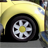Announcements
The way to achieve your own success is the willingness to help somebody else. Go for it!
cancel
Showing results for
Did you mean:Partner - Creator

## Hiding row if Condition

Hi,

I have the following image represented expression.

if(Column(1)>Column(2),'red.png',if(Column(1)=Column(2),'green.png','yellow.png'))

Basically, Column 1 and Column 2 contain numbers and I want to see if there is a difference between them by adding a colored smiley face on the right of each row. but now, I want to only reveal the rows where column 1 and column 2 are NOT equal to each other, meaning instead of showing the "green.png" I want to hide the whole row because I don't need to see it anymore.

How do i do this?

1 Solution

Accepted SolutionsPartner - Champion III

Hi

You will have to do this with the full expression from each column:

Column 1:

=If(<col1 expression> = <col2 expression>, <col1 expression>)

Column 2:

=If(<col1 expression> = <col2 expression>, <col2 expression>)

Where <col* expression> is the current expression in column *

HTH

Jonathan

Logic will get you from a to b. Imagination will take you everywhere. - A Einstein
3 RepliesPartner - Champion III

Hi

You will have to do this with the full expression from each column:

Column 1:

=If(<col1 expression> = <col2 expression>, <col1 expression>)

Column 2:

=If(<col1 expression> = <col2 expression>, <col2 expression>)

Where <col* expression> is the current expression in column *

HTH

Jonathan

Logic will get you from a to b. Imagination will take you everywhere. - A EinsteinSpecialist III

Dear ,

Can you attach the sample file.

Thanks,

MukramMVP

You need to hide while writing the expression itself..

Column1 Expression

IF(Column1 Expression <> Column2 Expression, Column1 Expression)

Column2 Expression

IF(Column1 Expression <> Column2 Expression, Column2 Expression)Community Browser